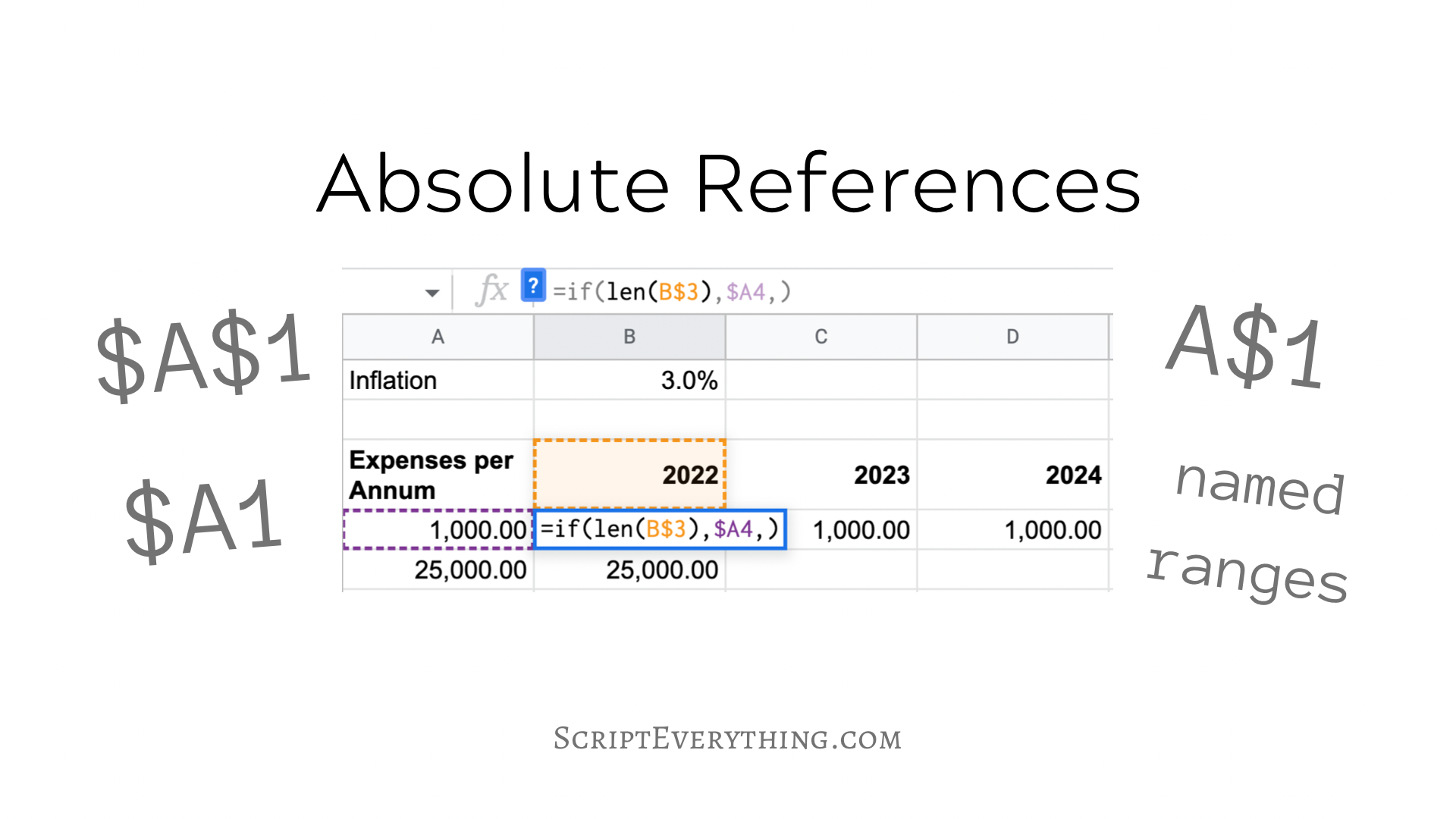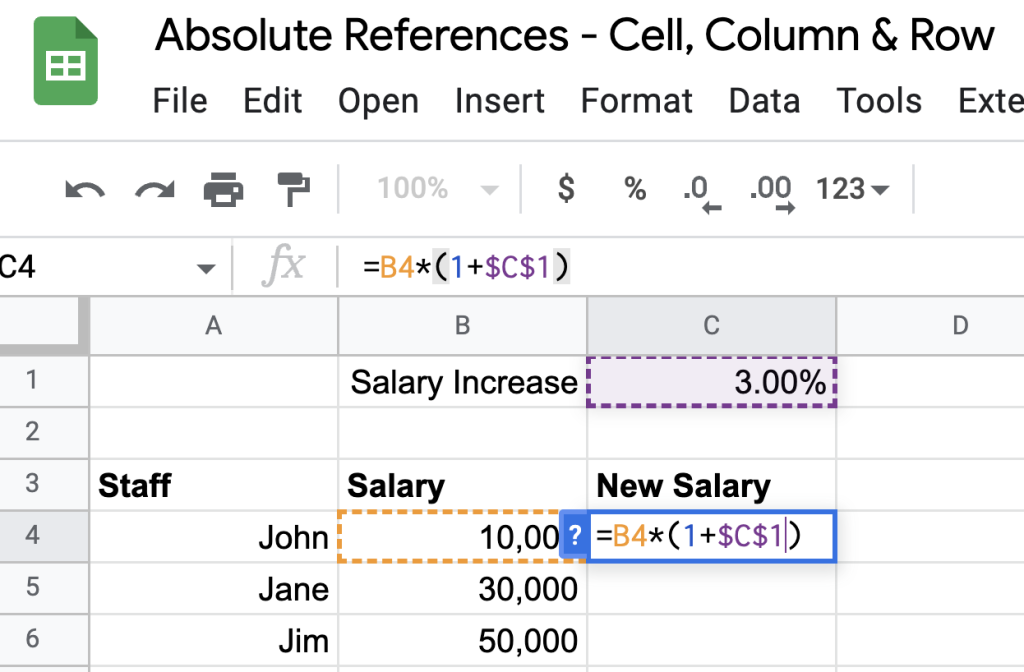# How To Use Absolute Cell References In Excel And Google SheetsHow do you use absolute cell references in a spreadsheet like Excel or Google Sheets?

An absolute cell reference is a reference containing the common column letter and row number labels but around those labels are either one or two dollar signs. For example, the following references for cell ``` A1 ``` are all absolute references: ``` \$A\$1 ``` , ``` \$A1 ``` and ``` A\$1 ``` .

What’s the difference between each of three references?

The purpose of the dollar sign in front of the column label and/or the row label is that this fixes the column or label. This helps when copying a cell and you want the reference of the column or row or cell to remain static .

Don’t get confused with other terms used with spreadsheets that contain the word absolute . For example, an absolute function and an absolute value have nothing to do with an absolute reference . The absolute function is the ``` =ABS() ``` formula which takes a number and returns its absolute value (a number without a negative sign).

While these terms have no relation with absolute references, an absolute function could take an absolute reference that would return an absolute value! (mind blown)

## Fix Cell Reference

The most common approach when using absolute references is where a formula is fixed to a specific cell. When designing your spreadsheet you may have a reference to a ``` salesTax ``` setting or some other important value that some cells reference but instead of copying the value everywhere you can just place it once and then perform an absolute reference to that cell.

A frequent use of an absolute cell reference is applying a salary increase or inflation increase on expenses. Here is a simple example where a salary increase of ``` 3.0% ``` is applied to each staff member:Cell C1 is the absolute cell and the formulas in column C reference this cell using the absolute annotation \$C\$1

As you can see from the above snapshot the formula in cell ``` C4 ``` to calculate the new salary for staff after their salary increase references the shared and common static value in ``` C1 ``` . As this same formula is used for all rows in column ``` C ``` to make the copying easier the cell ``` C1 ``` is made absolute by fixing the column and the row ``` \$C\$1 ``` .

When typing or clicking on the absolute cell to reference in your formula instead of having to type the absolute dollar signs you can use the shortcut key ``` F4 ``` . You can keep hitting the ``` F4 ``` key and the absolute reference will cycle through the different types: absolute cell ``` \$C\$1 ``` , absolute row ``` C\$1 ``` and absolute column ``` \$C1 ``` .

If you hit the ``` F4 ``` key ``` 4 ``` times it will remove all absolute references from the cell.

## Why Not Just Use A Named Range Instead?

Another way of using an absolute-like reference is to give the cell a name. If you have formulas referencing multiple absolute cells it can be difficult trying to track and ensure you’re referencing the right cell.

A way around this is to name your cell ranges with something meaningful. In my example, cell ``` C1 ``` is named ``` payRaise ``` .

To name a cell simply click on it and in the cell reference area (where you see the label ``` C1 ``` ) click on it and type the name you want to give that cell:

Then when referencing this range instead of using ``` \$C\$1 ``` you can use the alternative named range ``` payRaise ``` .

## Fix Row Reference

Besides fixing the column and the row labels by making an absolute cell reference you can opt to have the absolute part act on the row label only .

This means when you are copying a cell containing an absolute row reference that the row value will remain the same.

Here’s an example demonstrating an absolute row reference:

In the example above the first part of the formula ``` =if(len( B\$3 ),... ``` the reference in the ``` len ``` function contains an absolute row reference. This checks if a value exists in the header row containing the years.

An absolute row reference helps when you are copying cells down as the row label (the number) stays the same as you are copying the cell down.

As seen in the above spreadsheet snapshot, the formula contained in cell ``` B4 ``` is ``` =if(len(B\$3),\$A4,) ``` when this formula is copied down to cell ``` B5 ``` the formula in ``` B5 ``` is ``` =if(len(B\$3),\$A5,) ``` – notice here with the reference in the ``` len() ``` function that the column stayed the same and the row stayed the same too.

When the same original cell ``` B4 ``` is copied across to cell ``` C3 ``` the formula in ``` C3 ``` becomes ``` =if(len(C\$3),\$A4,) ``` – notice here with the reference in the ``` len() ``` function that the column value changed, but the row value did not.

This is how an absolute row reference works. When you copy an absolute cell down or across the row number in the copied cells will stay the same.

## Fix Column Reference

Using the same formula as expressed above you would have noticed in the ``` TRUE ``` parameter of the ``` IF ``` function that there was a reference where the column was fixed: ``` =if(len(B\$3), \$A3 ,) ``` .

By making the column reference absolute it helps when copying cells across .

Therefore, in cell ``` C4 ``` , as shown above, the formula keeps the column value static: ``` =if(len(C\$3),\$A4,) ``` . Notice how the reference ``` \$A4 ``` keeps the same column label, even though you are copying across (to the right).

When copying the cells down the row label will change, but the column label will not. Therefore, as seen above the cell in ``` B5 ``` is ``` =if(len(B\$3),\$A5,) ``` . Notice here the reference has changed to ``` \$A5 ``` where the column has stayed the same, but the row value has changed.

This is the nature of absolute column references . The column value remains the same when you are copying across or down.

## Summary

An absolute reference is a reference used in a cell that fixes either the column or the row or both. An absolute reference is seen by the symbol ``` \$ ``` (dollar sign) in front of the column and/or the row.

An absolute cell reference has both the column and row fixed and therefore will have the dollar symbol at the front of both the column label and the row label. For example, ``` \$A\$1 ``` is an absolute cell reference using cell ``` A1 ``` . Copying an absolute cell reference up, down or across will mean the reference stays the same.

An absolute row reference is where the row is fixed only and therefore will have the dollar symbol in front of just the row label. For example, ``` A\$1 ``` fixes the row to ``` 1 ``` . Copying an absolute row reference up or down will not change the reference, but copying the absolute row reference across will mean the row number stays the same and the column value will change.

An absolute column reference is where the column is fixed only and therefore will have the dollar symbol in front of the column label. For example, ``` \$A1 ``` fixes the column to ``` A ``` . Copying an absolute column reference across will not change the reference, but copying the absolute column reference up or down will mean the column stays the same and the row value will change.

Finally, instead of using an absolute cell reference, you could look at using named ranges instead. This might be helpful if you have many different absolute cell references in your spreadsheet.

Another type of reference used in spreadsheets is a relative reference . This is the opposite of an absolute reference but may come in handy when used in conditional formatting .

Author of scripteverything.com, Ryan has been dabbling in code since the late '90s when he cut his teeth by exploring VBA in Excel when trying to do something more. Having his eyes opened with the potential of automating repetitive tasks, he expanded to Python and then moved over to scripting languages such as HTML, CSS, Javascript and PHP. When he is not behind a screen, Ryan enjoys a good bush walk with the family during the cooler months, and going with them to the beach during the warmer months.